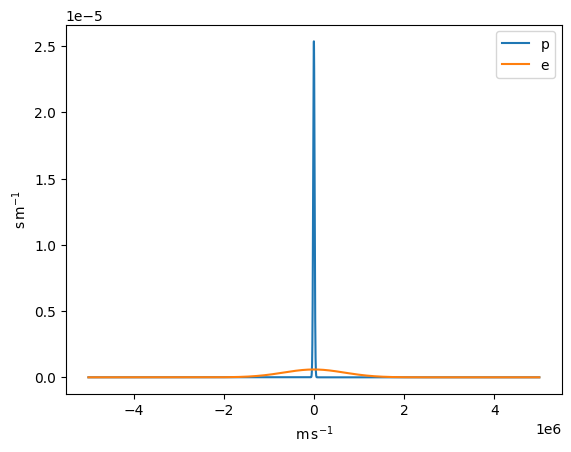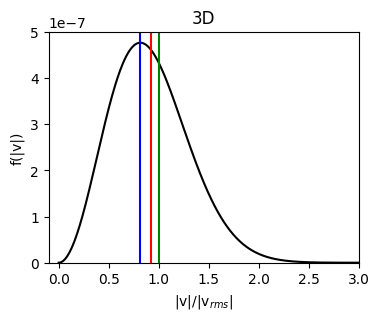# Distribution functions (plasmapy.formulary.distribution)

Common distribution functions for plasmas, such as the Maxwellian or Kappa distributions. Functionality is intended to include generation, fitting and calculation.

## Functions

 kappa_velocity_1D(v, T, kappa[, particle, ...]) Return the probability density at the velocity v in m/s to find a particle particle in a plasma of temperature T following the Kappa distribution function in 1D. kappa_velocity_3D(vx, vy, vz, T, kappa[, ...]) Return the probability density function for finding a particle with velocity components v_x, v_y, and v_zin m/s in a suprathermal plasma of temperature T and parameter kappa which follows the 3D Kappa distribution function. Maxwellian_1D(v, T[, particle, v_drift, ...]) Probability distribution function of velocity for a Maxwellian distribution in 1D. Maxwellian_speed_1D(v, T[, particle, ...]) Probability distribution function of speed for a Maxwellian distribution in 1D. Maxwellian_speed_2D(v, T[, particle, ...]) Probability distribution function of speed for a Maxwellian distribution in 2D. Maxwellian_speed_3D(v, T[, particle, ...]) Probability distribution function of speed for a Maxwellian distribution in 3D. Maxwellian_velocity_2D(vx, vy, T[, ...]) Probability distribution function of velocity for a Maxwellian distribution in 2D. Maxwellian_velocity_3D(vx, vy, vz, T[, ...]) Probability distribution function of velocity for a Maxwellian distribution in 3D.

## Examples